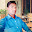# PETSc for Partial Differential Equations: Numerical Solutions in C and Python

· Software, Environments, and Tools Book 31 · SIAM
5.0
2 reviews
Ebook
407
Pages
Eligible

The Portable, Extensible Toolkit for Scientific Computation (PETSc) is an open-source library of advanced data structures and methods for solving linear and nonlinear equations and for managing discretizations. This book uses these modern numerical tools to demonstrate how to solve nonlinear partial differential equations (PDEs) in parallel. It starts from key mathematical concepts, such as Krylov space methods, preconditioning, multigrid, and Newton’s method. In PETSc these components are composed at run time into fast solvers.

Discretizations are introduced from the beginning, with an emphasis on finite difference and finite element methodologies. The example C programs of the first 12 chapters, listed on the inside front cover, solve (mostly) elliptic and parabolic PDE problems. Discretization leads to large, sparse, and generally nonlinear systems of algebraic equations. For such problems, mathematical solver concepts are explained and illustrated through the examples, with sufficient context to speed further development.

PETSc for Partial Differential Equations addresses both discretizations and fast solvers for PDEs, emphasizing practice more than theory. Well-structured examples lead to run-time choices that result in high solver performance and parallel scalability. The last two chapters build on the reader’s understanding of fast solver concepts when applying the Firedrake Python finite element solver library.

This textbook, the first to cover PETSc programming for nonlinear PDEs, provides an on-ramp for graduate students and researchers to a major area of high-performance computing for science and engineering. It is suitable as a supplement for courses in scientific computing or numerical methods for differential equations.

## Ratings and reviews

5.0
2 reviewsMITHILESH
September 24, 2021
Fantastic